# RD Sharma Class 10 Ex 11.2 Solutions Chapter 11 Constructions

In this chapter, we provide RD Sharma Class 10 Ex 11.2 Solutions Chapter 11 Constructions for English medium students, Which will very helpful for every student in their exams. Students can download the latest RD Sharma Class 10 Ex 11.2 Solutions Chapter 11 Constructions pdf, Now you will get step by step solution to each question.

# Chapter 11: Constructions​ Exercise – 11.2

### Question: 1

Draw an angle and label it as ∠BAC. Construct another angle, equal to ∠BAC.

### Solution:Steps of construction:

1. Draw an angle, ABO and a Line segment QR

2. With center A and any radius, draw an arc which intersects ∠BAC at E and O

3. With center Q and same radius draw arc which intersect QR at S.

4. With center S and radius equal to DE, draw an arc which intersect previous arc at T

5. Draw a line segment joining Q and T

∴ ∠PQR = ∠BAC

### ​Question: 2

Draw an obtuse angle, Bisect it. Measure each of the angles so obtained.

### Solution: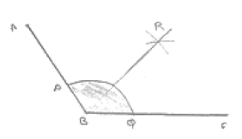Steps of construction:

1. Draw angle ABC of 120°

2. With center B and any radius, draw an arc which intersects AB at P and BC at Q

3. With center P and Q and radius more than 1/2 PQ, draw two arcs, with intersect each other at R.

4. Join BR

∴ ∠ABB = ∠RBC = 60°

### ​Question: 3

Using your protractor, thaw an angle of measure 108°. With this angle as given, draw an angle of 54°.

### Solution: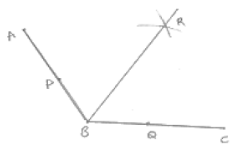Steps of construction:

1. Draw an angle ABC of 108°

2. With center B and any radius, draw an arc which intersects AB at P and BC at Q

3. With center P and Q and radius more than 2 PQ, draw two arcs, which intersect each

other at R.

4. Join BR

∴ ∠RBC = 54°

### ​Question: 4

Using protractor, draw a right angle. Bisect it to get an angle of measure 45°.

### Solution: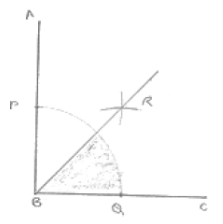Steps of construction:

1. Draw an angle ABC of 90°

2. With center B and any radius, draw an arc which intersects AB at P and BC at Q

3. With center P and Q and radius more than 1/2 PQ, draw two arcs, which intersect each 2 other at R.

4. Join RB

∴ ∠RBC = 45°

### ​Question: 5

Draw a linear pair of angles. Bisect each of the two angles. Verify that the two bisecting rays are perpendicular to each other.

### Solution:Steps of construction:

1. Draw two angle DCA and DCB forming Linear pair

2. With center C and any radius, draw an arc which intersects AC at P, CD at Q and CB at R.

3. With center P and Q and any radius draw two arcs which interest each other at S

4. Join SC

5. With center Q and R any radius draw two arcs, which intersect each other at T.

6. Join TC

∠SCT = 90° [By using protractor]

### ​Question: 6

Draw a pair of vertically opposite angles. Bisect each of the two angles. Verify that the bisecting rays are in the same line.

### Solution: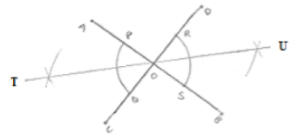Steps of construction:

1. Draw a pair of vertically opposite angle AOC and DOB

2. With center 0 and any radius drawn two arcs which intersect OA at P, Q – OB at S and OD at R.

3. With center P and Q and radius more than 1/2 PQ, draw two arcs which intersect each other at U.

4. Join to

5. With center R and S radius more than 1/2 RS, draw two arcs which intersect each other at U.

6. Join OU.

∴ TOU is a straight line

### ​Question: 7

Using ruler and compasses only, draw a right angle.

### Solution: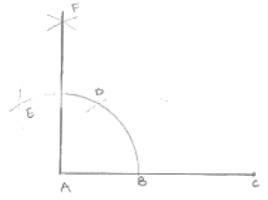Steps of construction:

1. Draw a line segment AB

2. With center A and any radius draw arc which intersect AB at C.

3. With center C and same radius draw an arc which intersects AB at C.

4. With center D and same radius draw arc which intersect arc in (2) at E.

5. With centers E and C and any radius, draw two arcs which intersect each other at F.

6. Join FA

∠FAB = 90°

### ​Question: 8

Using ruler and compasses only, draw an angle of measure 135°.

### Solution: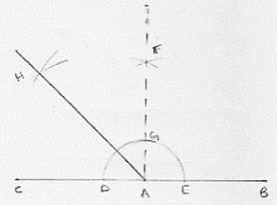Steps of construction:

1. Draw a line segment AB and produce BA to point C.

2. With center A and any radius draw arc which intersect AC at D and AB at E.

3. With center D and E and radius more than 1/2 DE, draw two arcs which intersect each other at F.

4. Join FA which intersect the arc in (2) at G.

5. With centers G and D and radius more than 1/2 GD, draw two arcs which intersect each other at H.

6. Join HA

∴ ∠HAB = 135°

### ​Question: 9

Using a protractor, draw an angle of measure 72°. With this angle as given, draw angles of measure 36° and 54°.

### Solution:

Steps of construction: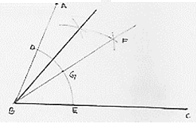1. Draw an angle ABC of 72° with the help of protractor.

2. With center B and any radius, draw an arc which intersect AB at D and BC at E.

3. With center D and E and radius more than 1/2 DE, draw two arcs which intersect each other at F.

4. Join FB which intersect the arc in (2) at G.

5. With centers D and G and radius more than 1/2 DE, draw two arcs which intersect each other at F.

6. With centers D and G and radius more than n 1/2 DG draw two arcs which intersect each other at H

7. Join HB

∴ ∠HBC = 54°

∠FBC = 36°

### ​Question: 10

Construct the following angles at the initial point of a given ray and justify the construction:

(i) 45°

(ii) 90°

### Solution: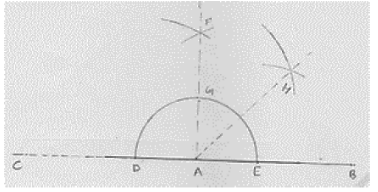(i) Steps of construction:

1. Draw a line segment AB and produce BA to point C.

2. With center A and any radius drawn an arc which intersect AC at D and AB at E.

3. With center D and E and radius more than 1/2 DE, draw arcs cutting each other at F.

4. Join FA which intersect arc in (2) at G.

5. With centers G and E and radius more than 1/2 GE, draw arcs cutting each other at H.

6. Join HA

∴ ∠HAB = 45°

(ii) Steps of construction: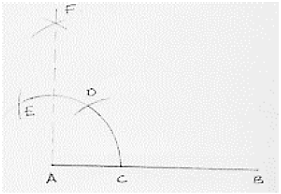1. Draw a line segment AB.

2. With center A and any radius draw in arc which intersect AB at C.

3. With center C and same radius thaw an arc which intersects previous arc at D.

4. With centers D same radius draw an arc which intersects are in (2) at E.

5. With centers E and D same radius more than 1/2 ED draw an arc cutting each other at F.

6. Join FA

∴ ∠FAB = 90°

All Chapter RD Sharma Solutions For Class10 Maths

I think you got complete solutions for this chapter. If You have any queries regarding this chapter, please comment on the below section our subject teacher will answer you. We tried our best to give complete solutions so you got good